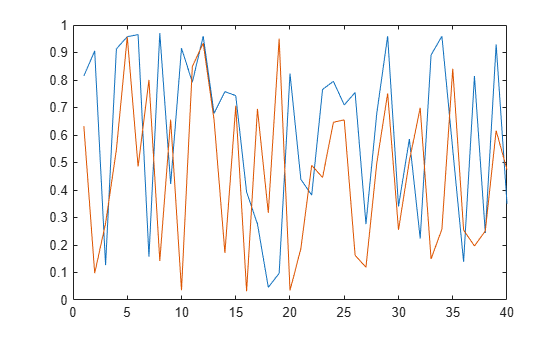# dsp.MatFileWriter

Write MAT file

## Description

The `dsp.MatFileWriter` System object™ writes data to a V7.3 MAT file.

To write data to a V7.3 MAT file:

1. Create the `dsp.MatFileWriter` object and set its properties.

2. Call the object with arguments, as if it were a function.

## Creation

### Syntax

``mfw = dsp.MatFileWriter``
``mfw = dsp.MatFileWriter(fname,vname)``
``mfw = dsp.MatFileWriter(Name,Value)``

### Description

````mfw = dsp.MatFileWriter` returns a MAT file writer System object, `mfw`, that writes data to a V7.3 MAT file.```

example

````mfw = dsp.MatFileWriter(fname,vname)` returns a MAT file writer System object with the `Filename` property set to `fname` and the `VariableName` property set to `vname`.```
````mfw = dsp.MatFileWriter(Name,Value)` returns a MAT file writer System object with each specified property set to the specified value. Unspecified properties have default values.```

## Properties

expand all

Unless otherwise indicated, properties are nontunable, which means you cannot change their values after calling the object. Objects lock when you call them, and the `release` function unlocks them.

If a property is tunable, you can change its value at any time.

Specify the name of a MAT file as a character vector or a string scalar. Specify the full path for the file only if the file is not on the MATLAB® path.

Name of the variable to which to write, returned as a character vector or a string scalar. This variable is stored in the MAT file. You cannot overwrite a variable that is already in an existing MAT file.

## Usage

### Syntax

``mfw(data)``

### Description

example

````mfw(data)` writes one frame of data to the variable stored in the MAT file. The variable is assumed to be N-dimensional and a MATLAB built-in data type. The data is written to the file by concatenating along the first dimension.```

### Input Arguments

expand all

Data to be written to the MAT file, specified as a scalar, vector, or a matrix. The data can be an N-dimensional array.

Data Types: `single` | `double` | `int8` | `int16` | `int32` | `int64` | `uint8` | `uint16` | `uint32` | `uint64`

## Object Functions

To use an object function, specify the System object as the first input argument. For example, to release system resources of a System object named `obj`, use this syntax:

`release(obj)`

expand all

 `step` Run System object algorithm `release` Release resources and allow changes to System object property values and input characteristics `reset` Reset internal states of System object

## Examples

collapse all

Note: This example runs only in R2016b or later. If you are using an earlier release, replace each call to the function with the equivalent `step` syntax. For example, myObject(x) becomes step(myObject,x).

First, create a variable name.

`filename = [tempname '.mat'];`

Next, write that variable to a MAT-file.

```mfw = dsp.MatFileWriter(filename,'VariableName','originalData'); for i = 1:10 originalData = rand(4,2); mfw(originalData); end release(mfw); % This will close the MAT file```

Finally, load the variable back into MATLAB.

`data = load(filename,'originalData');`

Plot the data.

`plot(data.originalData);`